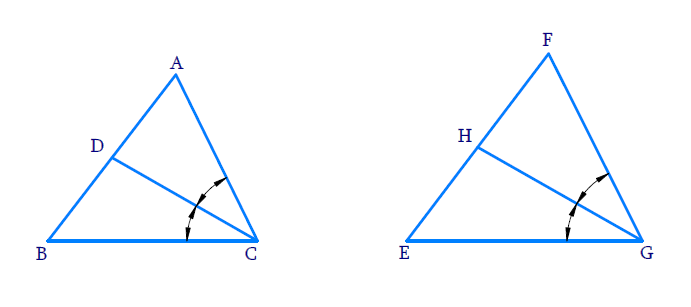# Ex.6.3 Q10 Triangles Solution - NCERT Maths Class 10

Go back to  'Ex.6.3'

## Question

$$CD$$ and $$GH$$ are respectively the bisectors of $$\angle ACB$$ and $$\angle EGF$$ such that $$D$$ and $$H$$ lie on sides $$AB$$ and $$FE$$ of $$\Delta ABC$$ and $$\Delta EFG$$ respectively. If $$\Delta ABC \sim \Delta FEG$$, show that:

(i)\begin{align}\frac{CD}{GH}=\frac{AC}{FG}\end{align}

(ii)\begin{align}\text{ }\Delta DCB\text{ }\sim{\ }\text{ }\Delta HGE\end{align}

(iii) \begin{align}\Delta DCA\text{ }\sim{\ }\Delta HGF\end{align}

DiagramVideo Solution
Triangles
Ex 6.3 | Question 10

## Text Solution

(i) Reasoning:

If two angles of one triangle are respectively equal to two angles of another triangle, then the two triangles are similar.

This may be referred to as the $$AA$$ similarity criterion for two triangles.

Steps:

$$\angle ACB\,=\,\angle FGE$$

\begin{align} \Rightarrow \frac {{\angle ACB}}{2}= \frac {{\angle FGE}}{2} \end{align}

$$\Rightarrow \angle ACD\,=\,\angle FGH$$ ($$CD$$ and $$GH$$ are bisectors of $$\angle C {\,\rm{and}} \,\angle G$$ respectively)

In $$\Delta ADC$$ and $$\Delta FHG$$

\begin{align}&\angle D A C=\angle H F G\\&[\because \Delta A D C \sim \Delta F E G]\\\\&\angle A C D=\angle F G H\\\Rightarrow \;&\Delta A D C\,\sim\,\Delta F H G\\& \;\;\text{(AA Criterion)} \end{align}

If two triangles are similar, then their corresponding sides are in the same ratio.

\begin{align} \Rightarrow \frac{C D}{G H}=\frac{A C}{F G}\end{align}

(ii) Reasoning:

If two angles of one triangle are respectively equal to two angles of another triangle,then the two triangles are similar.

This is reffered as AA criterion for two triangles.

Steps:

In $$\Delta DCB$$ and $$\Delta HGE$$

\begin{align}&\angle DBC=\angle HEG\,\\&\left[\because \Delta ABC\sim \Delta FEG \right] \\\\ &\angle DCB=\angle HGE\\&\left[\because \frac{\angle ACB}{2}=\frac{\angle FGE}{2} \right] \\\\\Rightarrow&\quad \Delta DCB \sim \Delta EHG\\&\quad\text{(AA Criterion}) \\ \end{align}

(iii) Reasoning:

If two angles of one triangle are respectively equal to two angles of another triangle,then the two triangles are similar.

This is reffered as AA criterion for two triangles.

Steps:

In $$\Delta DCA,\,\,\Delta HGF$$

\begin{align}& \angle DAC=\angle HFG\\&\left[ \because\Delta ABC\sim \Delta FEG \right] \\\\ & \angle ACD=\angle FGH\\&\left[\because \frac{{\angle ACB}}{2} = \frac {{\angle FGE}}{2}\right] \\\\ \Rightarrow\quad &\Delta DCA\sim \Delta HGF\\&\;\;\text{(AA Criterion}) \\ \end{align}

Learn from the best math teachers and top your exams

• Live one on one classroom and doubt clearing
• Practice worksheets in and after class for conceptual clarity
• Personalized curriculum to keep up with school